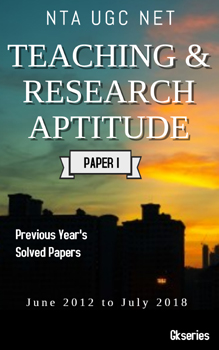# Psychology Questions and Answers for Competitive Exams | Psychology Quiz Set 61

Questions
1 We sometimes attempt to solve problems by applying the techniques that worked in similar situations in the past. What type of method are we using ?
1 Trial and error
2 Analogy
3 Algorithms
4 Heuristics

2 The fact that a nearer object may obscure a more distant object from the view is due to :
1 Figure and ground
2 Proximity
3 Interposition
4 Closure

3 In a 3 × 4 contingency table, using chi-square test, the degrees of freedom associated with the chi-square will be :
1 12
2 9
3 7
4 6

4 Read each of the following two statements – Assertion (A) andReason (R) and indicate your answerusing the codes below :

Assertion (A) : In Edwards Personal Preference Schedule (EPPS), forced choice format is used.

Reason (R) : Forced choice format reduces acquiescence response set.

#### codes:

1 Both (A) and (R) are true and (R) is the correct explanation of (A).
2 Both (A) and (R) are true but (R) is not the correct explanation of (A).
3 (A) is true but (R) is false.
4 (A) is false but (R) is true.

Answer:(A) is true but (R) is false.
5 Why do individuals with high need for achievement quit early when faced by very difficult task ?
1 Get tired easily
2 Have poor perseverance
3 Have high anxiety
4 Have high need for efficiency

6 Assertion (A) : According to R. B. Cattell, source traits are important in the understanding of personality.

Reason (R) : Oblique rotation is used for uncovering source traits.

#### Codes:

1 Both (A) and (R) are true and (R) is the correct explanation of (A).
2 Both (A) and (R) are true but (R)isnotthecorrect explanation of (A).
3 (A) is true but (R) is false.
4 (A) is false but (R) is true

Answer:Both (A) and (R) are true and (R) is the correct explanation of (A).
7 Which of the following represents the Tetrad equation as given by Spearman ?
1 r12 × r34 = r14 × r23
2 r13 × r24 = r14 × r23
3 r14 × r23 = r13 × r24
4 All of these

8 What among the following define a problem space ?

1. An initial state

2. A goal state

3. A set of operations

1 1, 3
2 1, 2
3 2, 3
4 1, 2, 3

9 A generalized picture or model of an object or event, learned in the past and stored in memory, is :
1 perceptual constancy
2 memory trace
3 prototype
4 cognitive map#### NTA UGC NET HISTORY E-BOOK – PREVIOUS YEAR’S SOLVED PAPERS(JUNE 2012 TO JULY 2018)#### NTA UGC NET ADULT EDUCATION E-BOOK – PREVIOUS YEAR’S SOLVED PAPERS(JUNE 2012 TO JULY 2018)#### NTA UGC NET ANTHROPOLOGY E-BOOK – PREVIOUS YEAR’S SOLVED PAPERS(JUNE 2012 TO JULY 2018)#### NTA UGC NET TEACHING & RESEARCH APTITUDE E-BOOK – PREVIOUS YEAR’S SOLVED PAPERS(JUNE 2012 TO JULY 2018)

10 Assertion (A) : We are able to recognize the similarity and differences of two objects.

Reason (R) : Dissociable subsystems operate simultaneously in each cerebral hemisphere.

#### Codes:

1 Both (A) and (R) are true, and (R) is the correct explanation of (A).
2 Both (A) and (R) are true, and (R) is not the correct explanation of (A).
3 (A) is true, but (R) is false.
4 Both (A) and (R) are false

Answer:Both (A) and (R) are true, and (R) is the correct explanation of (A).
11 What is the correct sequence of events given below ?

1. Releasing of neurotransmitters

2. Post synaptic potential

3. Binding on receptor sites

4. Synthesis of neurotransmitters

#### Codes:

1 1 2 3 4
2 4 3 2 1
3 4 1 3 2
4 2 4 1 3

12 Assertion (A) : The amplitude of the action potential in a neuron does not go beyond + 40 mV.

Reason (R) : Neurons function on “all or none” basis.

#### Codes:

1 Both (A) and (R) are true and (R) is the correct explanation of (A).
2 Both (A) and (R) are true, but (R) is not the correct explanation (A).
3 (A) is true, but (R) is false.
4 (A) is false, but (R) is true.

Answer:Both (A) and (R) are true, but (R) is not the correct explanation (A).
13 A man reflects his anger from his boss to his child, is an example of :
1 Projection
2 Reaction Formation
3 Displacement
4 Regression

14 Speed and power tests can be distinguished on the basis of :

1. Time limit

2. Difficulty level

3. Verbal/Non-verbal content

#### Codes:

1 1 and 2
2 1 and 3
3 2 and 3
4 1, 2 and 3

15 Natural concepts are based on :
1 prototypes
2 a set of rules/properties
3 schemas
4 propositions